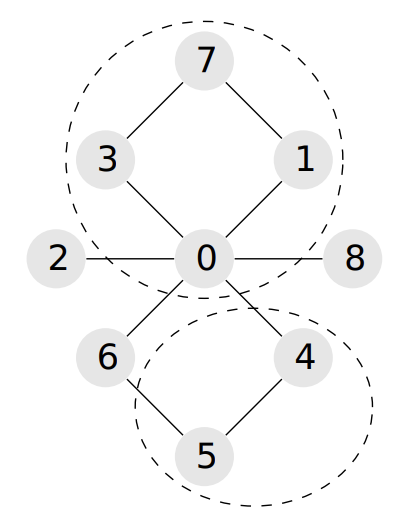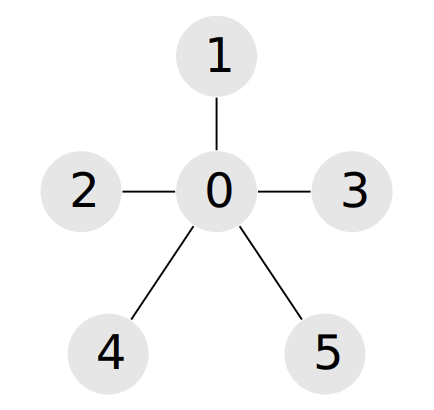시간 제한메모리 제한제출정답맞은 사람정답 비율
2 초 1024 MB42232365.714%

## 문제

There are $n$ attractions in Baku, numbered from $0$ to $n-1$. There are also $m$ two-way roads, numbered from $0$ to $m-1$. Each road connects two different attractions. It is possible to travel between any pair of attractions through the roads.

Fatima is planning to visit all of the attractions in three days. She already decided that she wants to visit $a$ attractions on the first day, $b$ attractions on the second day, and $c$ attractions on the third day. Therefore, she is going to partition the $n$ attractions into three sets $A$, $B$, and $C$ of sizes $a$, $b$, and $c$, respectively. Each attraction will belong to exactly one set, so $a + b + c = n$.

Fatima would like to find the sets $A$, $B$, and $C$, so that at least two out of the three sets are connected. A set $S$ of attractions is called connected if it is possible to travel between any pair of attractions in $S$ by using the roads and without passing through any attraction not in $S$. A partition of attractions into sets $A$, $B$, and $C$ is called valid if it satisfies the conditions described above.

Help Fatima find a valid partition of the attractions (given $a$, $b$, and $c$), or determine that no valid partition exists. If there are multiple valid partitions, you may find any of them.

## 구현

You should implement the following procedure:

int[] find_split(int n, int a, int b, int c, int[] p, int[] q)
• $n$: the number of attractions.
• $a$, $b$, and $c$: the desired sizes of sets $A$, $B$, and $C$.
• $p$ and $q$: arrays of length $m$, containing the endpoints of the roads. For each $i$ ($0 \leq i \leq m-1$), $p[i]$ and $q[i]$ are the two attractions connected by road $i$.
• This procedure should return an array of length $n$. Denote the array by $s$. If there is no valid partition, $s$ should contain $n$ zeros. Otherwise, for $0 \leq i \leq n-1$, $s[i]$ should be one of $1$, $2$, or $3$ to denote that attraction $i$ is assigned to set $A$, $B$, or $C$, respectively.

## 제한

• $3 \leq n \leq 100\,000$
• $2 \leq m \leq 200\,000$
• $1 \leq a, b, c \leq n$
• $a+b+c = n$
• There is at most one road between each pair of attractions.
• It is possible to travel between any pair of attractions through the roads.
• $0 \leq p[i], q[i] \leq n-1$ and $p[i] \neq q[i]$ for $0 \leq i \leq m - 1$

## 예시 1

Consider the following call:

find_split(9, 4, 2, 3, [0, 0, 0, 0, 0, 0, 1, 3, 4, 5],
[1, 2, 3, 4, 6, 8, 7, 7, 5, 6])A possible correct solution is $[1, 1, 3, 1, 2, 2, 3, 1, 3]$. This solution describes the following partition: $A=\{0, 1, 3, 7\}$, $B=\{4, 5\}$, and $C=\{2, 6, 8\}$. The sets $A$ and $B$ are connected.

## 예시 2

Consider the following call:

find_split(6, 2, 2, 2, [0, 0, 0, 0, 0], [1, 2, 3, 4, 5])No valid partition exists. Therefore, the only correct answer is $[0, 0, 0, 0, 0, 0]$.

## 서브태스크

번호 배점 제한
1 7

Each attraction is an endpoint of at most two roads.

2 11

$a = 1$

3 22

$m = n-1$

4 24

$n \leq 2500, m \leq 5000$

5 36

## 출처• 문제를 만든 사람: Alireza Farhadi, Saeed Seddighin

## 제출할 수 있는 언어

C++17, C++14, C++20, C++14 (Clang), C++17 (Clang), C++20 (Clang)

## 채점 및 기타 정보

• 예제는 채점하지 않는다.